# word2vec源码解析

• CBOW
• Skip-Gram
• Hierarchical Softmax
• Negative Sampling

• 预处理。即变量的声明，全局变量的定义等；
• 构建词库。即包含文本的处理，以及是否需要有指定词库等；
• 初始化网络结构。即包含CBOW模型和Skip-Gram模型的参数初始化，Huffman编码的生成等；
• 多线程模型训练。即利用Hierarchical Softmax或者Negative Sampling方法对网络中的参数进行求解；
• 最终结果的处理。即是否保存和以何种形式保存。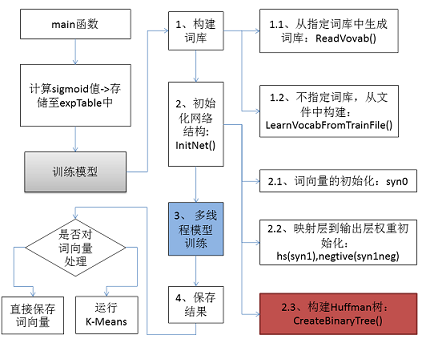# 1、预处理

$$\sigma \left ( x \right )=\frac{1}{1+e^{-x}}=\frac{e^x}{1+e^x}$$

expTable = (real *)malloc((EXP_TABLE_SIZE + 1) * sizeof(real));// 申请EXP_TABLE_SIZE+1个空间

// 计算sigmoid值
for (i = 0; i < EXP_TABLE_SIZE; i++) {
expTable[i] = exp((i / (real)EXP_TABLE_SIZE * 2 - 1) * MAX_EXP);  // Precompute the exp() table
expTable[i] = expTable[i] / (expTable[i] + 1);    // Precompute f(x) = x / (x + 1)
}

# 2、构建词库

• 从词的文本构建词库：LearnVocabFromTrainFile()方法

## 2.1、构建词库的过程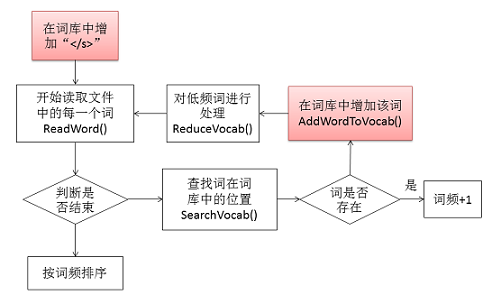if (ch == '\n') {
strcpy(word, (char *)"</s>");// 换行符用</s>表示
return;

## 2.2、对词的哈希处理

• 存储词的vocab
• 存储词的hash的vocab_hash

// 词的结构体
struct vocab_word {
long long cn; // 出现的次数
int *point; // 从根结点到叶子节点的路径
char *word, *code, codelen;// 分别对应着词，Huffman编码，编码长度
};

• 计算词的hash值：
// 取词的hash值
int GetWordHash(char *word) {
unsigned long long a, hash = 0;
for (a = 0; a < strlen(word); a++) hash = hash * 257 + word[a];
hash = hash % vocab_hash_size;
return hash;
}
• 检索词是否存在。如不存在则返回-1，否则，返回该词在词库中的索引：
while (1) {
if (vocab_hash[hash] == -1) return -1;// 不存在该词
if (!strcmp(word, vocab[vocab_hash[hash]].word)) return vocab_hash[hash];// 返回索引值
hash = (hash + 1) % vocab_hash_size;// 处理冲突
}
return -1;// 不存在该词

• 不存在，则插入新词。

## 2.4、根据词频对词库中的词排序

qsort(&vocab, vocab_size - 1, sizeof(struct vocab_word), VocabCompare);

# 3、初始化网络结构

## 3.1、初始化网络参数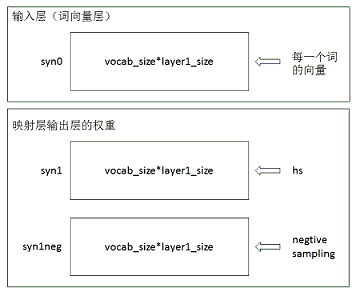for (a = 0; a < vocab_size; a++) for (b = 0; b < layer1_size; b++) {
next_random = next_random * (unsigned long long)25214903917 + 11;
// 1、与：相当于将数控制在一定范围内
// 2、0xFFFF：65536
// 3、/65536：[0,1]之间
syn0[a * layer1_size + b] = (((next_random & 0xFFFF) / (real)65536) - 0.5) / layer1_size;// 初始化词向量
}

## 3.2、Huffman树的构建

long long *count = (long long *)calloc(vocab_size * 2 + 1, sizeof(long long));
long long *binary = (long long *)calloc(vocab_size * 2 + 1, sizeof(long long));
long long *parent_node = (long long *)calloc(vocab_size * 2 + 1, sizeof(long long));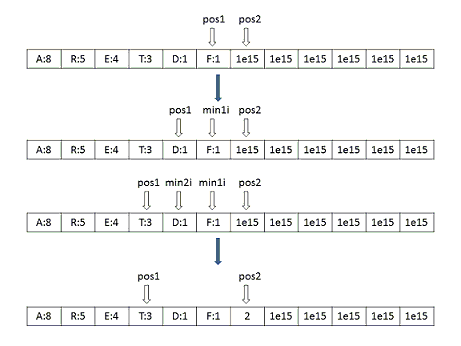// 设置父节点
parent_node[min1i] = vocab_size + a;
parent_node[min2i] = vocab_size + a;
binary[min2i] = 1;// 设置一个子树的编码为1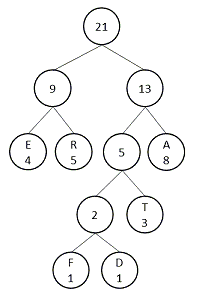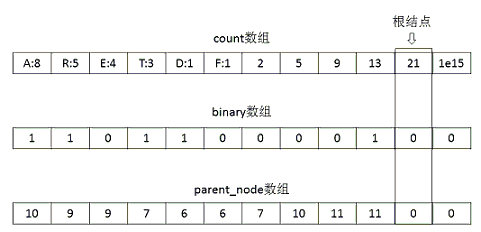vocab[a].codelen = i;// 词的编码长度
vocab[a].point = vocab_size - 2;
for (b = 0; b < i; b++) {
vocab[a].code[i - b - 1] = code[b];// 编码的反转
vocab[a].point[i - b] = point[b] - vocab_size;// 记录的是从根结点到叶子节点的路径
}

## 3.3、负样本选中表的初始化

// 生成负采样的概率表
void InitUnigramTable() {
int a, i;
double train_words_pow = 0;
double d1, power = 0.75;
table = (int *)malloc(table_size * sizeof(int));// int --> int
for (a = 0; a < vocab_size; a++) train_words_pow += pow(vocab[a].cn, power);
// 类似轮盘赌生成每个词的概率
i = 0;
d1 = pow(vocab[i].cn, power) / train_words_pow;
for (a = 0; a < table_size; a++) {
table[a] = i;
if (a / (double)table_size > d1) {
i++;
d1 += pow(vocab[i].cn, power) / train_words_pow;
}
if (i >= vocab_size) i = vocab_size - 1;
}
}

# 4、多线程模型训练

## 4.1、多线程的处理

// 利用多线程对训练文件划分，每个线程训练一部分的数据
fseek(fi, file_size / (long long)num_threads * (long long)id, SEEK_SET);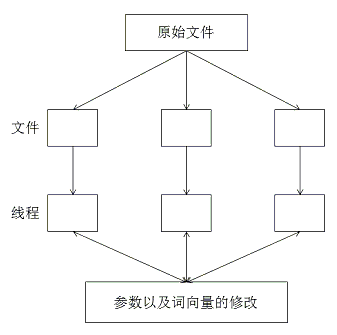## 4.2、CBOW模型

CBOW模型和Skip-gram模型是神经概率语言模型的两种变形形式，其中，在CBOW模型中包含三层，即输入层，映射层和输出层。对于CBOW模型，如下图所示：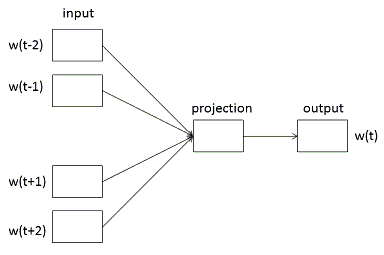### 4.3.1、从输入层到映射层

// in -> hidden
// 输入层到映射层
cw = 0;
for (a = b; a < window * 2 + 1 - b; a++) if (a != window) {
c = sentence_position - window + a;// sentence_position表示的是当前的位置
// 判断c是否越界
if (c < 0) continue;
if (c >= sentence_length) continue;

last_word = sen[c];// 找到c对应的索引
if (last_word == -1) continue;

for (c = 0; c < layer1_size; c++) neu1[c] += syn0[c + last_word * layer1_size];// 累加
cw++;
}

for (c = 0; c < layer1_size; c++) neu1[c] /= cw;// 计算均值

### 4.3.2、Hierarchical Softmax

Hierarchical Softmax是word2vec中用于提高性能的一项关键的技术。由Hierarchical Softmax的原理可知，对于词w，其对数似然函数为：

$$L\left ( w \right )=\sum _{j\in point}\left \{ \left ( 1-d_j^w \right )\cdot log\left [ \sigma \left ( X_w^T\theta _j^w \right ) \right ]+d_j^w\cdot log\left [ 1-\sigma \left ( X_w^T\theta _j^w \right ) \right ] \right \}$$

$$L\left ( w,j \right )=\left ( 1-d_j^w \right )\cdot log\left [ \sigma \left ( X_w^T\theta _j^w \right ) \right ]+d_j^w\cdot log\left [ 1-\sigma \left ( X_w^T\theta _j^w \right ) \right ]$$

$$\frac{\partial L\left ( w,j \right )}{\partial \theta _j^w}=\left [ 1-d_j^w-\sigma \left ( X_w^T\theta _j^w \right ) \right ]X_w$$

$$\frac{\partial L\left ( w,j \right )}{\partial X_w}=\left [ 1-d_j^w-\sigma \left ( X_w^T\theta _j^w \right ) \right ]\theta _j^w$$

$$\theta _j^w=\theta _j^w+\eta \left [ 1-d_j^w-\sigma \left ( X_w^T\theta _j^w \right ) \right ]X_w$$

for (d = 0; d < vocab[word].codelen; d++) {// word为当前词
// 计算输出层的输出
f = 0;
l2 = vocab[word].point[d] * layer1_size;// 找到第d个词对应的权重
// Propagate hidden -> output
for (c = 0; c < layer1_size; c++) f += neu1[c] * syn1[c + l2];// 映射层到输出层

if (f <= -MAX_EXP) continue;
else if (f >= MAX_EXP) continue;
else f = expTable[(int)((f + MAX_EXP) * (EXP_TABLE_SIZE / MAX_EXP / 2))];// Sigmoid结果

// 'g' is the gradient multiplied by the learning rate
g = (1 - vocab[word].code[d] - f) * alpha;
// Propagate errors output -> hidden
for (c = 0; c < layer1_size; c++) neu1e[c] += g * syn1[c + l2];// 修改映射后的结果
// Learn weights hidden -> output
for (c = 0; c < layer1_size; c++) syn1[c + l2] += g * neu1[c];// 修改映射层到输出层之间的权重
}

// hidden -> in
// 以上是从映射层到输出层的修改，现在返回修改每一个词向量
for (a = b; a < window * 2 + 1 - b; a++) if (a != window) {
c = sentence_position - window + a;
if (c < 0) continue;
if (c >= sentence_length) continue;
last_word = sen[c];
if (last_word == -1) continue;
// 利用窗口内的所有词向量的梯度之和来更新
for (c = 0; c < layer1_size; c++) syn0[c + last_word * layer1_size] += neu1e[c];
}

### 4.3.3、Negative Sampling

// 标记target和label
if (d == 0) {// 正样本
target = word;
label = 1;
} else {// 选择出负样本
next_random = next_random * (unsigned long long)25214903917 + 11;
target = table[(next_random >> 16) % table_size];// 从table表中选择出负样本
// 重新选择
if (target == 0) target = next_random % (vocab_size - 1) + 1;
if (target == word) continue;
label = 0;
}

$$l\left ( w \right )=\sigma \left ( X_w^T\theta ^w \right )\cdot \prod _{u\in {NEG\left ( w \right )}}\left [ 1- \sigma \left ( X_w^T\theta ^u \right )\right ]$$

$$L=log\; l\left ( w \right )=log\prod _{u\in {{w}\cup NEG\left ( w \right )}}\left \{ \left [ \sigma \left ( X_w^T\theta ^u \right ) \right ]^{L^w\left ( u \right )}\cdot \left [ 1- \sigma \left ( X_w^T\theta ^u \right )\right ]^{1-L^w\left ( u \right )} \right \}$$

$$\sum _{u\in {{w}\cup NEG\left ( w \right )}}\left \{ {L^w\left ( u \right )}\cdot log\left [ \sigma \left ( X_w^T\theta ^u \right ) \right ]+\left [ 1-L^w\left ( u \right ) \right ]\cdot log \left [ 1- \sigma \left ( X_w^T\theta ^u \right )\right ] \right \}$$

$$L\left ( w,u \right )={L^w\left ( u \right )}\cdot log\left [ \sigma \left ( X_w^T\theta ^u \right ) \right ]+\left [ 1-L^w\left ( u \right ) \right ]\cdot log \left [ 1- \sigma \left ( X_w^T\theta ^u \right )\right ]$$

$$\frac{\partial L\left ( w,u \right )}{\partial \theta ^u}=\left [ L^w\left ( u \right )- \sigma \left ( X_w^T\theta ^u \right )\right ]X_w$$

$$\frac{\partial L\left ( w,u \right )}{\partial X_w}=\left [ L^w\left ( u \right )- \sigma \left ( X_w^T\theta ^u \right )\right ]\theta ^u$$

if (f > MAX_EXP) g = (label - 1) * alpha;
else if (f < -MAX_EXP) g = (label - 0) * alpha;
else g = (label - expTable[(int)((f + MAX_EXP) * (EXP_TABLE_SIZE / MAX_EXP / 2))]) * alpha;

for (c = 0; c < layer1_size; c++) neu1e[c] += g * syn1neg[c + l2];
for (c = 0; c < layer1_size; c++) syn1neg[c + l2] += g * neu1[c];

## 4.3、Skip-gram模型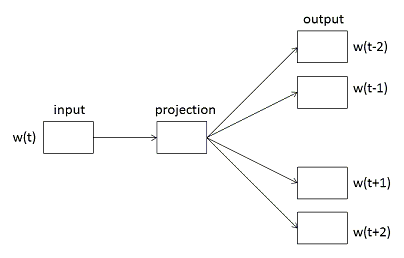### 4.3.1、Hierarchical Softmax

for (a = b; a < window * 2 + 1 - b; a++) if (a != window)

c = sentence_position - window + a;
if (c < 0) continue;
if (c >= sentence_length) continue;
last_word = sen[c];
if (last_word == -1) continue;
l1 = last_word * layer1_size;
for (c = 0; c < layer1_size; c++) neu1e[c] = 0;
// HIERARCHICAL SOFTMAX
if (hs) for (d = 0; d < vocab[word].codelen; d++) {
f = 0;
l2 = vocab[word].point[d] * layer1_size;
// Propagate hidden -> output
// 映射层即为输入层
for (c = 0; c < layer1_size; c++) f += syn0[c + l1] * syn1[c + l2];

if (f <= -MAX_EXP) continue;
else if (f >= MAX_EXP) continue;
else f = expTable[(int)((f + MAX_EXP) * (EXP_TABLE_SIZE / MAX_EXP / 2))];

// 'g' is the gradient multiplied by the learning rate
g = (1 - vocab[word].code[d] - f) * alpha;
// Propagate errors output -> hidden
for (c = 0; c < layer1_size; c++) neu1e[c] += g * syn1[c + l2];
// Learn weights hidden -> output
for (c = 0; c < layer1_size; c++) syn1[c + l2] += g * syn0[c + l1];
}
// Learn weights input -> hidden
for (c = 0; c < layer1_size; c++) syn0[c + l1] += neu1e[c];